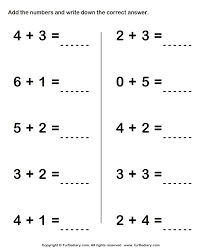# How Well Do You Know 1s And 10s

Approved & Edited by ProProfs Editorial Team
The editorial team at ProProfs Quizzes consists of a select group of subject experts, trivia writers, and quiz masters who have authored over 10,000 quizzes taken by more than 100 million users. This team includes our in-house seasoned quiz moderators and subject matter experts. Our editorial experts, spread across the world, are rigorously trained using our comprehensive guidelines to ensure that you receive the highest quality quizzes.
| By Jaksiboy
J
Jaksiboy
Community Contributor
Quizzes Created: 919 | Total Attempts: 12,132,600
Questions: 10 | Attempts: 163Settings. Certain principles apply in mathematics and this principle hasn’t change to date when given a set of equation to solve, taking and applying this principle gets the job done easily. Take a quiz using one of this principle of mathematics called addition and test your knowledge

• 1.

### The addition of five, plus three plus one will give us a number within the range of ten, what will the result be?

• A.

Nine

• B.

Seven

• C.

Nine

• D.

Five

C. Nine
Explanation
When we add five, plus three, plus one, we get a total of nine. This number falls within the range of ten, as stated in the question. Therefore, the result will be nine.

Rate this question:

• 2.

### Addition of two plus one, plus four will give a whole number within the range of ten, what exact figure will this be?

• A.

Five

• B.

Eight

• C.

Seven

• D.

Two

C. Seven
Explanation
Adding two plus one, plus four equals seven.

Rate this question:

• 3.

### Take the number five, add it to four, what number will this give within the range of ten, what is the answer?

• A.

Two

• B.

Nine

• C.

Seven

• D.

Three

B. Nine
Explanation
By taking the number five and adding it to four, we get a sum of nine. Since we are looking for a number within the range of ten, the only option that fits this criteria is nine. Therefore, the correct answer is nine.

Rate this question:

• 4.

### From the range of ten addition of certain number will give a final answer as six, what numbers can we add together to give us this?

• A.

Five plus two

• B.

One plus four

• C.

Nine plus zero

• D.

Three plus three

D. Three plus three
Explanation
The answer "Three plus three" is correct because when we add the numbers three and three together, the sum is six.

Rate this question:

• 5.

### Between zero to nine, what number can we add together and the Nine as the final result of the addition of these numbers?

• A.

Three plus five

• B.

Four plus five

• C.

Eight plus zero

• D.

Two plus six

B. Four plus five
Explanation
The numbers that can be added together to get a sum of nine are three and six, four and five, and eight and one. However, since the options only include numbers between zero and nine, the only option that satisfies this condition is four plus five.

Rate this question:

• 6.

### Addition of zero plus one plus zero plus 3 plus zero plus one will give the final result within the range of ten, what will the result be?

• A.

Three

• B.

Four

• C.

Two

• D.

Five

D. Five
Explanation
When we add zero plus one plus zero plus three plus zero plus one, we get a total of five. Therefore, the final result will be five.

Rate this question:

• 7.

### Take seven, add it to one plus zero plus one plus zero will give the final result within the range of ten, the final answer is?

• A.

Ten

• B.

Nine

• C.

Four

• D.

Two

B. Nine
Explanation
Adding seven to one plus zero plus one plus zero equals nine.

Rate this question:

• 8.

### Take a particular number and add it to another plus another and the final result will be five, which number do we need to add together?

• A.

Three plus two plus five

• B.

Eight plus zero plus two

• C.

One plus four plus one

• D.

Zero plus three plus two

D. Zero plus three plus two
Explanation
If we add zero, three, and two together, the sum will be five.

Rate this question:

• 9.

### The addition of seven plus two plus zero will give certain final result within the range of ten, what is the final answer?

• A.

Seven

• B.

Five

• C.

Nine

• D.

Ten

C. Nine
Explanation
The addition of seven plus two plus zero equals nine, which falls within the range of ten. Therefore, the final answer is nine.

Rate this question:

• 10.

### Three plus zero plus one plus two plus four plus zero will give a certain final figure within one to ten, what is the final answer?

• A.

Five

• B.

Eight

• C.

Ten

• D.

NineBack to top